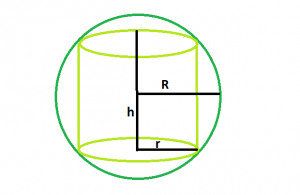# Volume of largest right circular cylinder within a Sphere

Given a sphere of radius. The task is to find volume of the biggest right circular cylinder that can be inscribed within it.

Examples:

```Input : R = 4
Output : 77.3495

Input : R = 5
Output : 151.073
```

## Recommended: Please try your approach on {IDE} first, before moving on to the solution.Approach:
let r be the radius of the right circular cylinder, and h be it’s height.

Volume of the cylinder, V = π*r2*h

Also, r2 = R2 – h2
or, V = π*(R2 – h2)*h
or, dV/dh = π*(R2 – 3*h2)

Setting it to zero, we get h = R/√3
So, Vmax = 2πR3/3√3

Below is the implementation of the above approach:

## C++

 `// C++ Program to find the biggest right circular cylinder ` `// that can be fit within a sphere ` ` `  `#include ` `using` `namespace` `std; ` ` `  `// Function to find the biggest right circular cylinder ` `float` `cyl(``float` `R) ` `{ ` ` `  `    ``// radius cannot be negative ` `    ``if` `(R < 0) ` `        ``return` `-1; ` ` `  `    ``// volume of cylinder ` `    ``float` `V = (2 * 3.14 * ``pow``(R, 3)) / (3 * ``sqrt``(3)); ` `    ``return` `V; ` `} ` ` `  `// Driver code ` `int` `main() ` `{ ` `    ``float` `R = 4; ` ` `  `    ``cout << cyl(R) << endl; ` ` `  `    ``return` `0; ` `} `

## Java

 `// Java Program to find the biggest ` `// right circular cylinder that can ` `// be fit within a sphere ` `import` `java.io.*; ` ` `  `class` `GFG ` `{ ` ` `  `// Function to find the biggest ` `// right circular cylinder ` `static` `float` `cyl(``float` `R) ` `{ ` ` `  `    ``// radius cannot be negative ` `    ``if` `(R < ``0``) ` `        ``return` `-``1``; ` ` `  `    ``// volume of cylinder ` `    ``float` `V = (``float``)((``2` `* ``3.14` `* Math.pow(R, ``3``)) /  ` `                      ``(``3` `* Math.sqrt(``3``))); ` `    ``return` `V; ` `} ` ` `  `// Driver code ` `public` `static` `void` `main (String[] args) ` `{ ` `    ``float` `R = ``4``; ` ` `  `    ``System.out.print( cyl(R)); ` `} ` `} ` ` `  `// This code is contributed by anuj_67..  `

## Python 3

 `# Python 3 Program to find the biggest  ` `# right circular cylinder that can be ` `# fit within a sphere ` `import` `math ` ` `  `# Function to find the biggest right  ` `# circular cylinder ` `def` `cyl(R): ` `     `  `    ``# radius cannot be negative ` `    ``if` `(R < ``0``): ` `        ``return` `-``1` ` `  `    ``# volume of cylinder ` `    ``V ``=` `((``2` `*` `3.14` `*` `math.``pow``(R, ``3``)) ``/`  `                ``(``3` `*` `math.sqrt(``3``))); ` `    ``return` `float``(V) ` ` `  `# Driver code ` `R ``=` `4` `print``(cyl(R)) ` ` `  `# This code is contributed ` `# by PrinciRaj1992 `

## C#

 `// C# Program to find the biggest ` `// right circular cylinder that can ` `// be fit within a sphere ` `using` `System; ` ` `  `class` `GFG ` `{ ` ` `  `// Function to find the biggest ` `// right circular cylinder ` `static` `float` `cyl(``float` `R) ` `{ ` ` `  `    ``// radius cannot be negative ` `    ``if` `(R < 0) ` `        ``return` `-1; ` ` `  `    ``// volume of cylinder ` `    ``float` `V = (``float``)((2 * 3.14 * Math.Pow(R, 3)) /  ` `                             ``(3 * Math.Sqrt(3))); ` `    ``return` `V; ` `} ` ` `  `// Driver code ` `public` `static` `void` `Main () ` `{ ` `    ``float` `R = 4; ` ` `  `    ``Console.WriteLine( cyl(R)); ` `} ` `} ` ` `  `// This code is contributed by shs  `

## PHP

 ` `

Output:

```77.3495
```

Attention reader! Don’t stop learning now. Get hold of all the important DSA concepts with the DSA Self Paced Course at a student-friendly price and become industry ready.

My Personal Notes arrow_drop_upCheck out this Author's contributed articles.

If you like GeeksforGeeks and would like to contribute, you can also write an article using contribute.geeksforgeeks.org or mail your article to contribute@geeksforgeeks.org. See your article appearing on the GeeksforGeeks main page and help other Geeks.

Please Improve this article if you find anything incorrect by clicking on the "Improve Article" button below.